• +91 9971497814
• info@interviewmaterial.com

# Chapter 8- Gravitation Interview Questions Answers

### Related Subjects

Question 1 :
(a) You can shield a charge from electrical forces by putting it inside a hollow conductor. Can you shield a body from the gravitational influence of nearby matter by putting it inside a hollow sphere or by some other means?
(b) An astronaut inside a small space ship orbiting around the earth cannot detect gravity. If the space station orbiting around the earth has a large size, can he hope to detect gravity?
(c) If you compare the gravitational force on the earth due to the sun to that due to the moon, you would find that the Sun’s pull is greater than the moon’s pull. (You can check this yourself using the data available in the succeeding exercises). However, the tidal effect of the moon’s pull is greater than the tidal effect of sun. Why?

(a) Gravitational influence of matter on nearby objects cannot be screened by any means. This is because gravitational force unlike electrical forces is independent of the nature of the material medium. Also, it is independent of the status of other objects.
(b) If the size of the space station is large enough, then the astronaut will detect the change in Earth’s gravity (g).
(c) Tidal effect depends inversely upon the cube of the distance while, gravitational force depends inversely on the square of the distance. Since the distance between the Moon and the Earth is smaller than the distance between the Sun and the Earth, the tidal effect of the Moon’s pull is greater than the tidal effect of the Sun’s pull.

Question 2 :

Choose the correctalternative:

(a) Acceleration due togravity increases/decreases with increasing altitude.

(b) Acceleration due togravity increases/decreases with increasing depth. (assume the earth to be asphere of uniform density).

(c) Acceleration due togravity is independent of mass of the earth/mass of the body.

(d) The formula –G Mm(1/r2– 1/r1) is more/less accuratethan the formula mg(r2– r1) for the difference ofpotential energy between two points r2and r1distance away from thecentre of the earth.

(a) Decreases

(b) Decreases

(c) Mass of the body

(d) More

Explanation:

(a) Acceleration due togravity at depth h is given by the relation: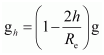Where,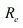= Radius of theEarth

g = Acceleration due togravity on the surface of the Earth

It is clear from the givenrelation that acceleration due to gravity decreases with an increase in height.

(b) Acceleration due togravity at depth d is given by the relation: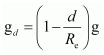It is clear from the givenrelation that acceleration due to gravity decreases with an increase in depth.

(c) Acceleration due to gravity of body ofmass m is given by the relation: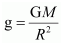Where,

G = Universalgravitational constant

M = Mass of the Earth

Hence, it can be inferredthat acceleration due to gravity is independent of the mass of the body.

(d) Gravitationalpotential energy of two points r2 and r1 distance away fromthe centre of the Earth is respectively given by: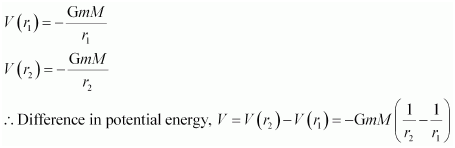Hence,this formula is more accurate than the formula mg(r2– r1).

Question 3 :

Supposethere existed a planet that went around the sun twice as fast as the earth.Whatwould be its orbital size as compared to that of the earth?

Answer: Lesser by a factorof 0.63

Time taken by the Earth tocomplete one revolution around the Sun,

Te = 1 year

Orbital radius of the Earth in itsorbit, R= 1 AU

Timetaken by the planet to complete one revolution around the Sun,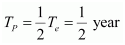Orbital radius of the planet = Rp

From Kepler’s third law ofplanetary motion, we can write: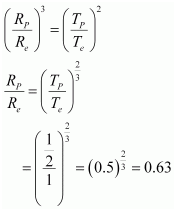Hence,the orbital radius of the planet will be 0.63 times smaller than that of theEarth.

Question 4 :

Io, one of the satellites of Jupiter, has anorbital period of 1.769 days and the radius of the orbit is 4.22 × 108 m. Show that themass of Jupiter is about one-thousandth that of the sun.

Answer 4 : Orbital period of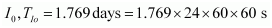Orbital radius of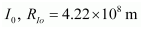Satellite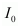is revolving around theJupiter

Mass of the latter isgiven by the relation: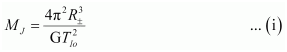Wre,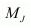= Mass of Jupiter

G = Universalgravitational constant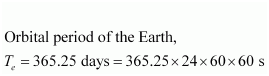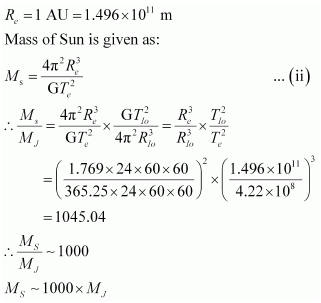Hence,it can be inferred that the mass of Jupiter is about one-thousandth that of theSun.

Question 5 :

Let us assume that our galaxy consists of2.5 × 1011 stars each of one solar mass. How longwill a star at a distance of 50,000 ly from the galactic centre take tocomplete one revolution? Take the diameter of the Milky Way to be 105 ly.

Mass of our galaxy Milky Way, M =2.5 × 1011 solar mass

Solar mass = Mass of Sun = 2.0 × 1036 kg

Mass of our galaxy, M = 2.5× 1011 × 2 × 1036 = 5 ×1041 kg

Diameter of Milky Way, d =105 ly

Radius of Milky Way, r = 5× 104 ly

1 ly = 9.46 × 1015 m

r = 5 × 104 × 9.46 × 1015

= 4.73 ×1020 m

Since a star revolvesaround the galactic centre of the Milky Way, its time period is given by therelation: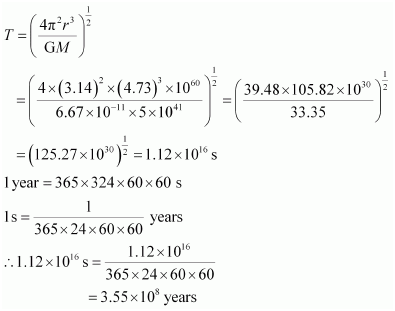Question 6 :

Choose the correctalternative:

(a) If the zero of potentialenergy is at infinity, the total energy of an orbiting satellite is negative ofits kinetic/potential energy.

(b) The energy required tolaunch an orbiting satellite out of earth’s gravitational influence ismore/less than the energy required to project a stationary object at the sameheight (as the satellite) out of earth’s influence.

(a) Kinetic energy

(b) Less

(a) Total mechanicalenergy of a satellite is the sum of its kinetic energy (always positive) andpotential energy (may be negative). At infinity, the gravitational potentialenergy of the satellite is zero. As the Earth-satellite system is a boundsystem, the total energy of the satellite is negative.

Thus, the total energy ofan orbiting satellite at infinity is equal to the negative of its kineticenergy.

(b)An orbiting satellite acquires a certain amount of energy that enables it torevolve around the Earth. This energy is provided by its orbit. It requiresrelatively lesser energy to move out of the influence of the Earth’sgravitational field than a stationary object on the Earth’s surface thatinitially contains no energy.

Question 7 :
Does the escape speed of a body from the earth depend on
(a) the mass of the body,
(b) the location from where it is projected,
(c) the direction of projection,
(d) the height of the location from where the body is launched?

a) No

(b) No

(c) No

(d) Yes

Escape velocity of a bodyfrom the Earth is given by the relation: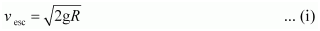g = Acceleration due togravity

R = Radius of the Earth

Itis clear from equation (i) that escape velocity vesc is independent of the massof the body and the direction of its projection. However, it depends ongravitational potential at the point from where the body is launched. Sincethis potential marginally depends on the height of the point, escape velocityalso marginally depends on these factors.

Question 8 : A comet orbits the Sun in a highly elliptical orbit. Does the comet have a constant (a) linear speed, (b) angular speed, (c) angular momentum, (d) kinetic energy, (e) potential energy, (f) total energy throughout its orbit? Neglect any mass loss of the comet when it comes very close to the Sun.

(a) No
(b) No
(c) Yes
(d) No
(e) No
(f) Yes
Angular momentum and total energy at all points of the orbit of a comet moving in a highly elliptical orbit around the Sun are constant. Its linear speed, angular speed, kinetic, and potential energy varies from point to point in the orbit.

Question 9 : Which of the following symptoms is likely to afflict an astronaut in space (a) swollen feet, (b) swollen face, (c) headache, (d) orientational problem?

(a) Legs hold the entire mass of a body in standing position due to gravitational pull. In space, an astronaut feels weightlessness because of the absence of gravity. Therefore, swollen feet of an astronaut do not affect him/her in space.
(b) A swollen face is caused generally because of apparent weightlessness in space. Sense organs such as eyes, ears nose, and mouth constitute a person’s face. This symptom can affect an astronaut in space.
(c) Headaches are caused because of mental strain. It can affect the working of an astronaut in space.
(d) Space has different orientations. Therefore, orientational problem can affect an astronaut in space.

Question 10 :
Choose the correct answer from among the given ones:
The gravitational intensity at the centre of a hemispherical shell of uniform mass density has the direction indicated by the arrow (see Fig 8.12) (i) a, (ii) b, (iii) c, (iv) O.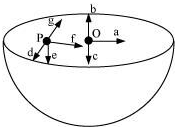Gravitational potential (V) is constant at all points in a spherical shell. Hence, the gravitational potential gradient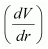is zero everywhere inside the sphericalshell. The gravitational potential gradient is equal to the negative ofgravitational intensity. Hence, intensity is also zero at all points inside thespherical shell. This indicates that gravitational forces acting at a point ina spherical shell are symmetric.

If the upper half of aspherical shell is cut out (as shown in the given figure), then the netgravitational force acting on a particle located at centre O will be in thedownward direction.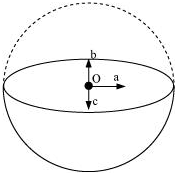Sincegravitational intensity at a point is defined as the gravitational force perunit mass at that point, it will also act in the downward direction. Thus, thegravitational intensity at centre O of the given hemispherical shell has thedirection as indicated by arrow c.

Todays Deals### Chapter 8- Gravitation Contributorskrishan

Name:
Email:

# Latest News# 9000 interview questions in different categories